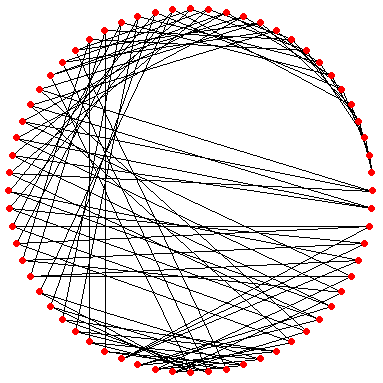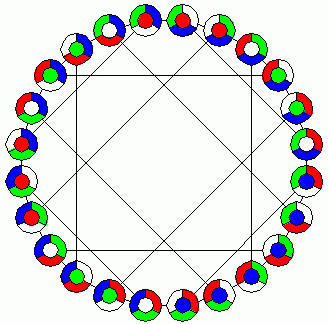Math Games Cubic Symmetric Graphs Ed Pegg Jr., December 30, 2003

In my Domino Graphs column, I mentioned the Petersen Graph. There, I looked at all the dominoes formed by choosing two numbers from 0 1 2 3 4. Each domino connects to exactly three others, so the graph can be considered cubic -- each node has exactly three edges. The graph is also symmetric -- any of the ten dominoes could be at the center (try it!). The set of words {wiz, two, bet, pub, lip, sly, son, gun, zag, aye} makes the Petersen graph in a different way (how?). Another symmetric graph involves taking the disjoint triples of a 7-set to form the Odd graph O4. Take 3 different consonants from D L N P R S T in all 35 possible ways. Add vowels to create words, then delete this heptad of words: plus, surd, land, pirn, nest, dept, terl. The resulting object is the Coxeter graph, shown below. Words salt and pond don't share consonants, so they are connected.Words lint and trap share a T, so they are not connected. I used red for T, so I colored the connecting edge for sped and lorn red, since it's the odd man out.Figure 1. The Petersen Graph and the Coxeter Graph.

Pick your favorite word in the Coxeter graph, and travel around until you get back to your chosen word, without reusing any edges. If your cycle has length 7, you will find that you used seven differently colored paths. (That's the shortest possible cycle, so the Coxeter graph has girth 7). If your cycle has length 8, the edges use 4 colors, each doubled. If your cycle has length 9, you used all seven colors, one tripled. Isn't that amazing? What color combinations happens with cycles of other lengths? From any word, you can reach any other word within 4 steps, so the Coxeter graph has a diameter of 4. Famed geometer HSM Coxeter was a great lover of symmetry -- he liked this graph so much that he wrote a paper about it called simply My Graph. Figure 2 shows different isomorphisms of his graph. If you have a spare set of dominoes laying around, you might find it an enjoyable task to attach the 28 words, and then to plunk them down on each isomorphism. The first two versions are three colored. Notice how every vertex has three different colors. Most cubic graphs can be three-colored -- rare exceptions like the Petersen graph are called snarks. I leave the three-colorings of the other two versions as an exercise.Figure 2. Different versions of the Coxeter Graph, some three-colored.

When I first started looking at these graphs, I learned of the Foster Census, which is a listing of all of the cubic symmetric graphs up to 768 vertices.  Here's an example of one of them, F64. So far, all the graphs I've shown have had a variety of symmetrical-looking pictures.  I'm certain F64 has a nice picture, but I haven't found it yet.  For almost a year, F90 -- also called the Foster Graph -- was a minor obsession of mine.  I wanted to see it. I moved dots and lines around in all sorts of different ways. I learned many tricks -- computer searches, symmetry finding, line crossings, and hamiltonian paths. Someday, I'll find a nice F64.Figure 3. F64 in it's raw form.  Puzzle: Make it pretty.

In Figure 1, the Petersen graph's paths cross each other 6 times, and the paths of the Coxeter graph cross each other 15 times. The number of crossings is aptly named the crossing number.  Geoff Exoo has a page showing famous graphs with minimized crossing numbers. The Petersen graph can be drawn with just 2 crossings, and the Coxeter graph with just 11. The Heawood graph can be drawn with 3 crossings. Connect the dots in the figure below with straight lines to illustrate these minimal-crossing representations.Figure 4. Connect the dots with straight lines to make a Petersen graph and Heawood graph. Answer.

Here are pictures of various cubic symmetric graphs. I found F56A by moving nodes around on my computer screen. I found the outside circle first, and then moved the other nodes close to them.  Suddenly, I saw this very striking figure. I was hooked.Figure 5. Various Cubic Symmetric Graphs

In 1980, Roberto Frucht, HSM Coxeter, and David Powers developed a method for describing hundreds of zero-symmetric graphs. In the symmetric graphs described on this page, any vertex or edge is equivalent to any other vertex or edge.  In a zero-symmetric graph, the vertices are equivalent, but the edges are maximally dissimilar.  I call their graph description method the Frucht notation. The cubic graph (F8) can be represented as [3,-3]4 in Frucht notation. The graph starts with a hamiltonian cycle on the outside.  After that, the vertices are connected by going clockwise 3 spaces, then counterclockwise 3 spaces -- do this 4 times.  F14 is [5,-5]7, F16 is [5,-5]8, F38 is [15,-15]19. The raw form of F38 is somewhat long, so you can see why I started liking Frucht notations. F18 is [5, 7, -7, 7, -7, -5]3.  F60 is a bit complicated: [12, -17, -12, 25, 17, -26, -9, 9, -25, 26]6.Figure 6. Cubic Symmetric Graphs with Frucht notations

Many of these graphs are important enough to have specific names. See Eric Weisstein's page on Symmetric Cubic Graphs for a list. One graph that probably deserves a name is F24. Get three different small objects, and put them on 4 different sheets of paper.  You may move any object to the empty sheet.  There are 24 different configurations.  When all the connections are drawn, F24 results.Figure 7. F24, as a diagram of 3 colored marbles.

After many different computer searches, I hit on [17, -9, 37, -37, 9, -17]15 --it's the Foster Graph (F90).  I finally got a chance to see it.  I'm glad I searched.Figure 8. F90 - The Foster Graph

References:

Coxeter, HSM., Frucht, R., Powers, D. Zero-symmetric graphs. Academic Press, 1981.

Coxeter, HSM. "My graph". Proc. London Math. Soc. (3) 46 (1983) 117-136.

Royle, G. Algebraic Graph Theory. Springer, 2001.

Royle, G.  The Foster Census. http://www.cs.uwa.edu.au/~gordon/remote/foster/.  Originally published in 1934 by Ronald M Foster.

Weisstein, E. Symmetric Cubic Graph.  Eric Weisstein's World of Mathematics. http://mathworld.wolfram.com/SymmetricCubicGraph.html

Wolfram, S. A New Kind of Science. Wolfram Media, Inc., Champaign Il, 2002. p 1032.

Many thanks to Stephen Wolfram for asking me to draw F4 to F28, and to Eric Weisstein in maintaining interest in the graphs beyond.

Mathematica Code:

Many of the page at mathworld.wolfram.com have extensive notebooks. http://mathworld.wolfram.com/SymmetricCubicGraph.html is one of those pages.  See also his extensive packages on graph theory at http://library.wolfram.com/infocenter/MathSource/4775/. Anyone who desires my code for Frucht Notation searches, or who finds any, should write me at the address below.

Comments are welcome. Please send comments to Ed Pegg Jr. at ed@mathpuzzle.com.

Ed Pegg Jr. is the webmaster for mathpuzzle.com. He works at Wolfram Research, Inc. as the administrator of the Mathematica Information Center.